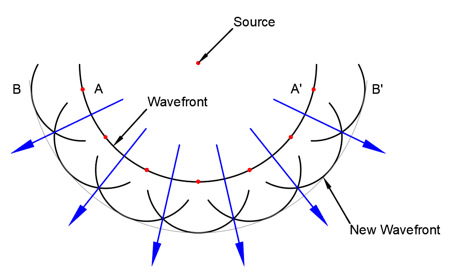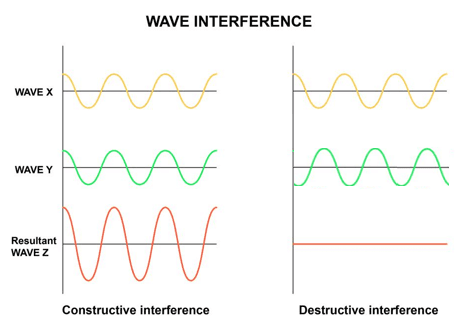## Lesson 10 – Light Wave Interference

Posted on: Friday, April 29th, 2022  In: Learning Optics with Austin

Besides explaining rainbows, why model light as a wave? The particle model of light is usually drawn with a particle traveling as a ray. Just by staring at a candle or light bulb however, we know that light travels in all directions. It would be impossible to draw out infinite rays traveling in all directions, so the wave model is the solution for where the particle model falls short. Instead of drawing out infinite rays of light, a wave is composed of infinite particles that are in the same phase. Each of these particles then goes on to emit their own wavefront, which is the idea behind Huygens’ Principle. As shown in the diagram below, Huygens’ Principle complements the particle model of light as rays can be drawn where the wavefronts cross.Huyghen’s Diagram

The overlapping wavefronts naturally lead to the question of what happens when two light waves meet each other. If the following three conditions are met, then interference will take place:
1) If they have the same frequency
2) If they have the same direction of vibration
3) If the phase difference is constantSoap Bubble

A simple example of interference is the multi-colored surface of a soap bubble. The colorful surface is produced by a phenomenon known as thin-film interference. The science behind interference itself has a lot to do with the constructive and destructive effects of waves in general. When two light waves are in phase with each other, constructive interference results in a greater intensity of light. When light waves are out of phase with each other, destructive interference results and the light waves cancel each other (more on that in the next post!).Constructive and Destructive Interference

Interference might seem to contradict the assumption early on that light paths are independent of one another (see “Diffraction and Refraction” post). This is not the case however, because the path of light is not being changed when two light waves meet. Light neither bounces off nor merges together. Instead, light continues on its path but either becomes brighter or dimmer depending on the interaction of light waves. While light waves are too small for the human eye to detect, interference can be demonstrated by water waves.Water Waves

There are ways however, in which interference can be measured and studied. Read more in depth on interference in the upcoming post!

Next: Lesson 11 – Fringes and Interferometers